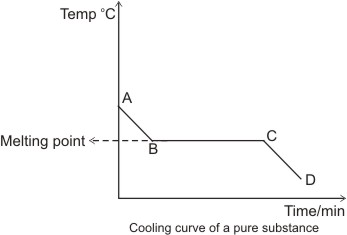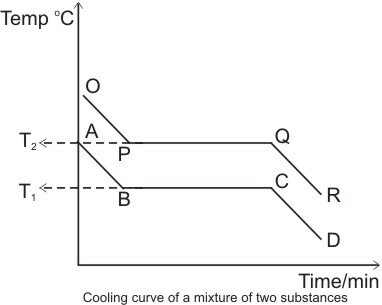## The Cooling Curve

When a solid substance is heated until it is completely melted, and then heated further and allowed to cool slowly, the curve obtained by plotting temperature against time is known as the cooling curve.Explanations for the cooling curve above:

Between A and B, the temperature falls with time - there is no change of state, hence the substance is still in the liquid state.

Between B and C, there is a constant temperature over time. This suggests that there is a change of state going on from liquid to solid. The heat lost between these points is that gained in liquefying the solid substance (latent heat of fusion). Hence, there is a balance of heat, which explains why there is no reading of temperature change- this steady temperature is the melting point of the substance and the section, B and C represents liquid/solid state.

Between C and D, the temperature falls again with time. Here, the substance is totally in the solid state.

The cooling curve discussed above is that of a pure solid substance. If the substance were a mixture (i.e. consisting of two or more components), we would have more than one level of steady temperature. This is because we would have different melting points for the different components present. Example:T1 and T2 are the melting points of the two components of the mixture respectively.

Note: the melting point or freezing point is the temperature at which the solid and liquid phases of a substance can exist in equilibrium. At this point, the solid and liquid phases have the same vapor pressure.

 Like This Post? Please Share!

Copyright , All Rights Reserved Free Chemistry Online | About Us | Usage of Content | Total Disclosures | Privacy Policy